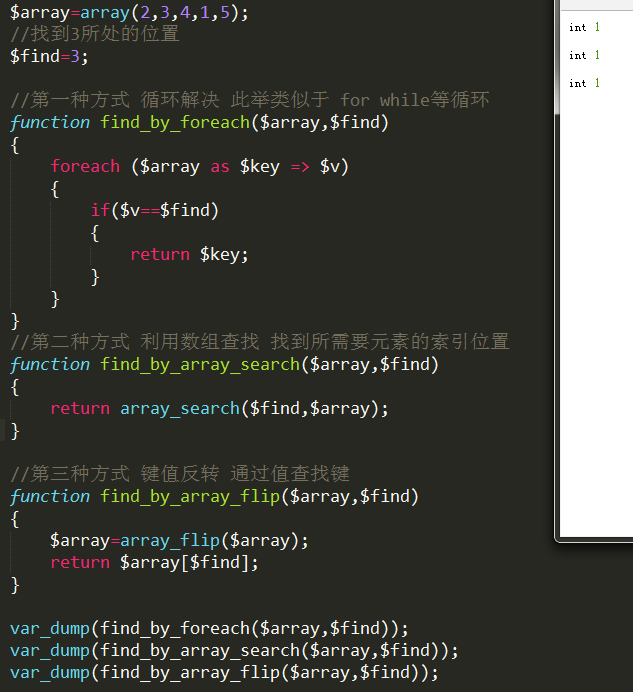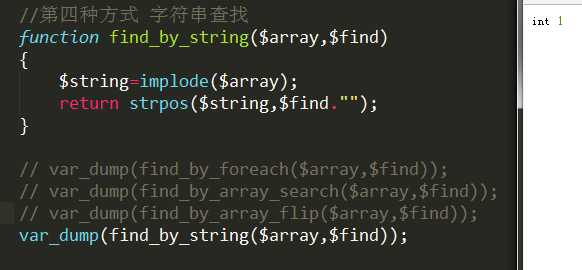# PHP从数组中找到指定元素的位置

2018/05/23 17:40

群里有人问，有个数组五个元素 分为1到5  现在要求 循环找出3元素的索引，怎么做性能才是最高。

我不知道哪个性能最高，但是我想提出可以用多种方式进行查找，然后进行比较选择。

array_flip键值反转，能够将你需要的键变值，值变键，然后取数组下标，即可得到这个位置。

<?php
$array=array(2,3,4,1,5); //找到3所处的位置$find=3;

//第一种方式 循环解决 此举类似于 for while等循环
function find_by_foreach($array,$find)
{
foreach ($array as$key => $v) { if($v==$find) { return$key;
}
}
}
//第二种方式 利用数组查找 找到所需要元素的索引位置
function find_by_array_search($array,$find)
{
return array_search($find,$array);
}

//第三种方式 键值反转 通过值查找键
function find_by_array_flip($array,$find)
{
$array=array_flip($array);
return $array[$find];
}

var_dump(find_by_foreach($array,$find));
var_dump(find_by_array_search($array,$find));
var_dump(find_by_array_flip($array,$find));<?php
//第四种方式 字符串查找
function find_by_string($array,$find)
{
$string=implode($array);
return strpos($string,$find."");
}0
0 收藏

### 作者的其它热门文章0 评论
0 收藏
0